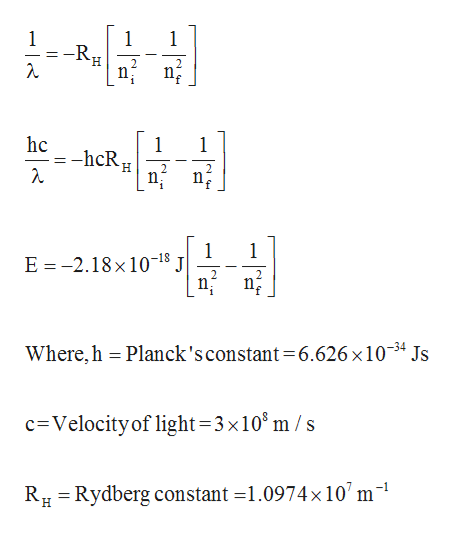# Calculate the energy for the transition of an electron from the n = 7 level to the n = 5 level of a hydrogen atom.E =_______JoulesIs this an Absorption (A) or an Emission (E) process ?

Question
1 views

Calculate the energy for the transition of an electron from the n = 7 level to the n = 5 level of a hydrogen atom.

E =_______Joules

Is this an Absorption (A) or an Emission (E) process ?

check_circle

Step 1

From Rydberg equation, the energy of electron transition can be calculated as given below.help_outlineImage Transcriptionclose1 -RH 1 1 hc 1 1 -hcR 2 n 1 E -2.18x 10-18 1 2 2 n Where,h Planck's constant =6.626 x 10-34 Js c Velocity of light=3x105 m / s Rydberg constant =1.0974x 107 m R H fullscreen
Step 2

Here, ni is 7 and nf is 5. Now, putting all the values in the above energy equati...

### Want to see the full answer?

See Solution

#### Want to see this answer and more?

Solutions are written by subject experts who are available 24/7. Questions are typically answered within 1 hour.*

See Solution
*Response times may vary by subject and question.
Tagged in

### Physical Chemistry